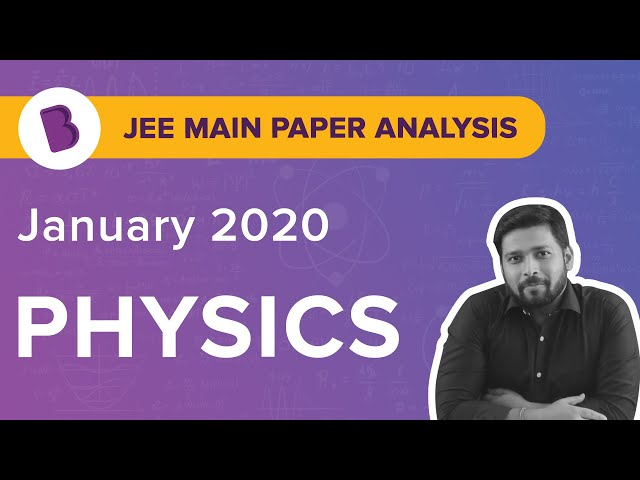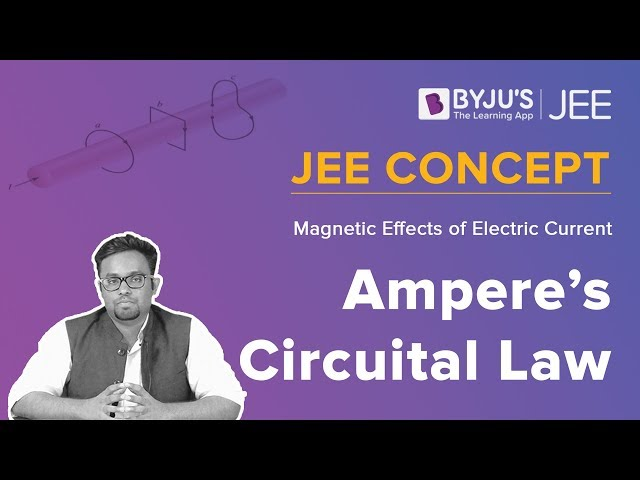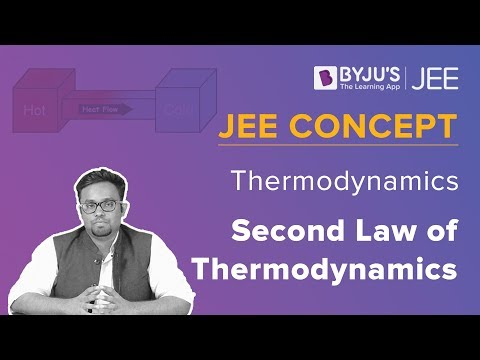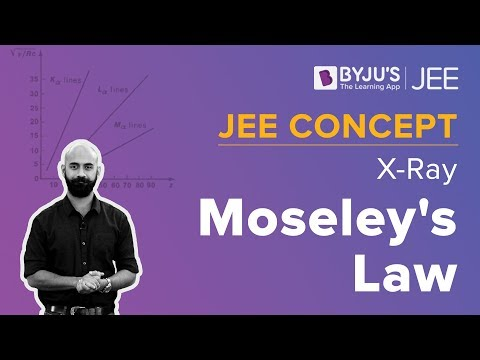Win up to 100% scholarship on Aakash BYJU'S JEE/NEET courses with ABNAT Win up to 100% scholarship on Aakash BYJU'S JEE/NEET courses with ABNAT

# JEE Main 2018 Physics Paper With Solutions Shift 1 Jan 10

JEE Main is an examination conducted for admission into some of the reputed colleges in India. Students can find the solved paper of JEE Main 2018 Physics Jan 10 Shift 1 on this page. The subject experts at BYJU’S have solved all these questions. These questions are answered in the easiest possible method. Students can also download these solutions from our website in PDF format.
Test
January 10 Shift 1 – Physics
1. It is found that if a neutron suffers an elastic collinear collision with deuterium at rest, fractional oss of its energy is pd; while for its similar collision with carbon nucleus at rest, fractional loss of energy is pC. The values of pd and pc are respectively:
a. (0, 0)
b. (0, 1)
c. (.89,.28)
d. (.28,.89)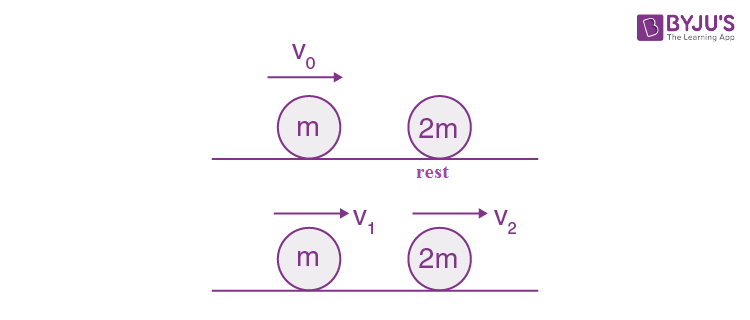L.M.C

mV1 + 2m V2 = mV0

V1 + 2V2 = V0 …(1)

$$\begin{array}{l}e=\frac{V_{2}-V_{1}}{V_{0}}=1\end{array}$$

V2 – V1 = V0…(2)

On solving

V2 = 2V0/3 and V1= V0/3

Final KE of neutron= (1/2)mV12= (1/2)m(V0/3)2

=

$$\begin{array}{l}\frac{1}{9}\left ( \frac{1}{2}mV_{0}^{2} \right )\end{array}$$

Loss of K.E =

$$\begin{array}{l}\frac{8}{9}\left ( \frac{1}{2}mV_{0}^{2} \right )\end{array}$$

Fractional loss Pd = (8/9) = 0.89

Similarly collision between N and C

mV1 + 12 m.V2 = mV0

V1 + 12V2 = V0 …(1)

V1-V2 = V0 …(2)

On Solving

V2 = 2V0/13

And V1 = 11V0/13

Final K.E =

$$\begin{array}{l}=\frac{1}{2}m\left [ \frac{11V_{0}^{2}}{13} \right ] = \frac{121}{169}\left [ \frac{1}{2}mV_{0}^{2} \right ]\end{array}$$

Loss of KE =

$$\begin{array}{l}= \frac{48}{169}\left [ \frac{1}{2}mV_{0}^{2} \right ]\end{array}$$

Fractional loss Pc = (48/169) = 0.28

2. The mass of a hydrogen molecule is 3.32 x 10-27 kg. If 1023 hydrogen molecules strike, per second, a fixed wall of area 2 cm2 at an angle of 450 to the normal, and rebound elastically with a speed of 103 m/s, then the pressure on the wall is nearly:
a. 2.35 x 102 N/m2
b. 4.70 x 102 N/m2
c. 2.35 x 103 N/m2
d. 4.70 x 103 N/m2

Change in momentum of one molecule

$$\begin{array}{l}\Delta P_{1}=2mvcos45^{0}=\sqrt{2}mv\end{array}$$

Force

$$\begin{array}{l}F= \frac{\Delta P}{\Delta t}=n \times \Delta P_{1}\end{array}$$

Where n -> no. of molecules incident per unit time

Pressure P = Force/Area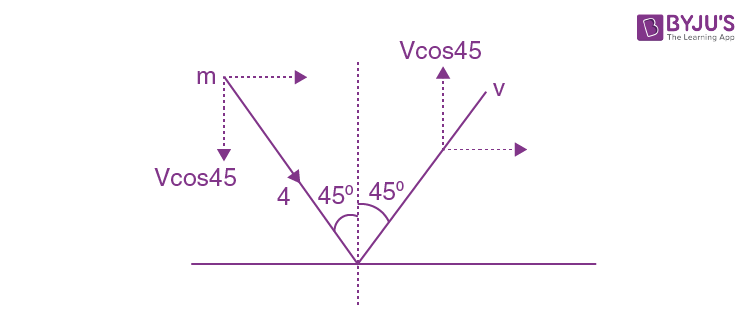$$\begin{array}{l}P = \frac{n\times \sqrt{2}mv}{A}\end{array}$$
$$\begin{array}{l}P = \frac{10^{23}\times \sqrt{2}\times 3.32\times 10^{-27}\times 10^{3}}{2\times 10^{-4}}\end{array}$$
$$\begin{array}{l}P = \frac{3.32}{1.41}\times 10^{3}=2.35 \times 10^{3}N/m^{2}\end{array}$$

3. A solid sphere of radius r made of a soft material of bulk modulus K is surrounded by a liquid in a cylindrical container. A mass less piston of area a floats on the surface of the liquid, covering entire cross section of cylindrical container. When a mass m is placed on the surface of the piston to compress the liquid, the fractional decrement in the radius of the sphere,(dr/r) is:
a. mg/3Ka
b. mg/Ka
c. Ka/mg
d. Ka/3mgBulk modulus

$$\begin{array}{l}K=\frac{-dP}{\frac{dV}{V}}\end{array}$$
$$\begin{array}{l}\frac{dV}{V}=\frac{dP}{K}\end{array}$$
$$\begin{array}{l}\frac{dV}{V}=\frac{mg}{Ka}\end{array}$$
——-(1)

$$\begin{array}{l}V=\frac{4}{3}\pi r^{3}\end{array}$$
$$\begin{array}{l}\frac{dV}{V}=3\frac{dr}{r}\end{array}$$
———(2)

From eq.(1) and (2)

$$\begin{array}{l}\frac{dr}{r}=\frac{mg}{3Ka}\end{array}$$

4. Two batteries with e.m.f. 12 V and 13 V are connected in parallel across a load resistor of 10 Ω. The internal resistances of the two batteries are 1 Ω and 2 Ω respectively. The voltage across the load lies between.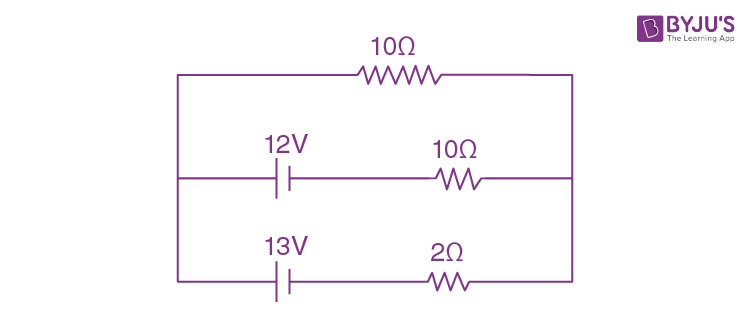a. 11.4 V and 11.5 V
b. 11.7 V and 11.8 V
c. 11.6 V and 11.7 V
d. 11.5 V and 11.6 V

$$\begin{array}{l}E_{eq}=\frac{\frac{E_{1}}{r_{1}}+\frac{E_{2}}{r_{2}}}{\frac{1}{r_{1}}+\frac{1}{r_{2}}}\end{array}$$
$$\begin{array}{l}E_{eq}=\frac{\frac{12}{1}+\frac{13}{2}}{\frac{1}{1}+\frac{1}{2}}=\frac{37}{3}\end{array}$$
$$\begin{array}{l}r_{eq}=\frac{1\times 2}{1+2}=\frac{2}{3}\Omega\end{array}$$
$$\begin{array}{l}i=\frac{E_{eq}}{R+r_{eq}}-\frac{\frac{37}{3}}{10+\frac{2}{3}}=\frac{37}{32}amp\end{array}$$
$$\begin{array}{l}\Delta V =iR = \frac{37}{32}\times 10=\frac{185}{16}=11.56\end{array}$$

5. A particle is moving in a circular path of radius a under the action of an attractive potential U = -k/2r2. Its total energy is
a. zero
b.
$$\begin{array}{l}\frac{-3k}{2a^{2}}\end{array}$$

c.
$$\begin{array}{l}\frac{-k}{4a^{2}}\end{array}$$

d.
$$\begin{array}{l}\frac{k}{2a^{2}}\end{array}$$

$$\begin{array}{l}U =\frac{-k}{2r^{2}}\end{array}$$
$$\begin{array}{l}F =\frac{-du}{dr}=+\frac{k}{2}\left ( \frac{-2}{r^{3}} \right )\end{array}$$

F= -k/r3

Centripetal force

$$\begin{array}{l}\frac{mv^{2}}{r}=\frac{k}{r^{3}}\end{array}$$
$$\begin{array}{l}mv^{2}=\frac{k}{r^{2}}\end{array}$$
$$\begin{array}{l}KE= \frac{1}{2}mv^{2}=\frac{k}{2r^{2}}\end{array}$$

Total Energy E = K + U = 0

6. Two masses m1 = 5 kg and m2 = 10 kg, connected by an inextensible string over a frictionless pulley, are moving as shown in the figure. The coefficient of friction of horizontal surface is 0.15. The minimum weight m that should be put on top of m2 to stop the motion is: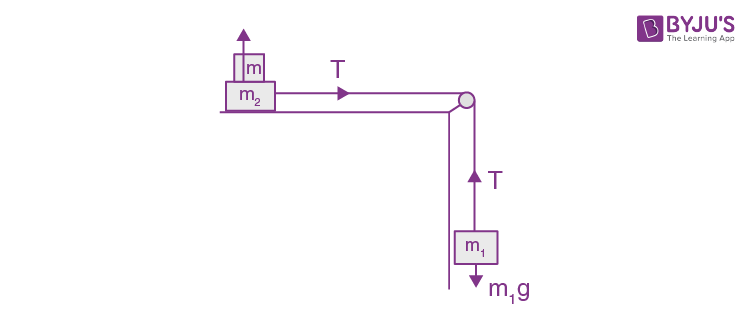a. 43.3 Kg
b. 10.3 Kg
c. 18.3 Kg
d. 27.3 Kg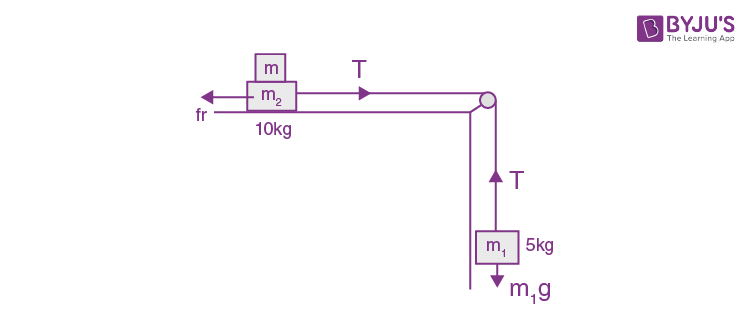At equilibrium fr = T = m1g

frmax =(m2 + m)g

(10+m)g =5g

10 +m = 5/0.15

10+m = 100/3

m = (70/3)kg = 23.3 kg.

The minimum weight in the options is 27.3 kg.

7. If the series limit frequency of the Lyman series is vL, then the series limit frequency of the Pfund series is:
a. vL/16
b. vL/25
c. 25vL
d. 16vL

Series limit is

Lyman : ∞

→ 1

P fund :∞
→ 5

vLyman = RC

vPfund = RC/25

8. Unpolarized light of intensity I passes through an ideal polarizer A. Another identical polarizer B is placed behind A. the intensity of light beyond B is found to be I/2. Now another identical polarizer C is placed between A and B. The intensity beyond B is now found to be I/8. The angle between polarizer A and C is:
a. 450
b. 600
c. 00
d. 300

Unpolarized light passes the A

I after A = I/2

I after B = I/2 (given)

The angle between A and B is 900

Let A and C have angle θ

I after c = (I/2)cos2 θ

I after B = (I/2)cos2 θ cos2(90 – θ )= I/8, therefore, [cos θ sin θ]2= ¼

Sin 2 θ = 1

Therefore, θ = 450

9. An electron from various excited states of hydrogen atom emit radiation to come to the ground state. Let λn, λg be the de Broglie wavelength of the electron in the nth state and the ground state respectively. Let An be the wavelength of the emitted photon in the transition from the nth state to the ground state. For large n, (A, B are constants)
a. Λ2n≈ A+B λ2n
b. Λ2n≈ λ
c. Λn≈A + (B/ λ2n)
d. Λn≈A + Bλn

mvr = (nh/2 π)

λde Broglie = (h/p) = (2 πr/n)

λn =(2 πrn/n)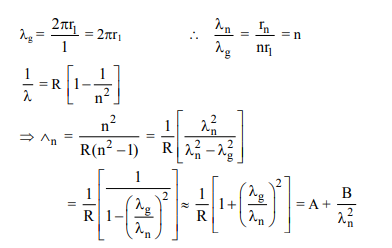10. The reading of the ammeter for a silicon diode in the given circuit is: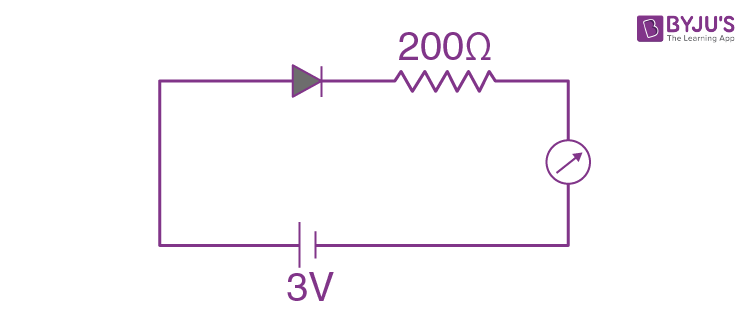a. 11.5 mA
b. 13.5 mA
c. 0
d. 15 mA

Knowledge based

Si diode has forward bias resistance

200 at 2 V

400 at 1 V

⇒ here Rfb < 200 … at 3V

I = (V-ΔV)/R = (3-0.7)/200 = 2.3/200 = 11.5 mA

11. An electron, a proton and an alpha particle having the same kinetic energy are moving in circular orbits of radii re, rp, rα respectively in a uniform magnetic field B. The relation between re, rp, rα is:
a. re < rp < rα
b. re < rα < rp
c. re > rp = rα
d. re < rp = rα

r = mv/qB = p/qB

K = (1/2)mv2——-same

= (p2/2m)

Therefore, p ∞ √m

B same

r∝(p/q) or √m/q

qp =qe

mp = 183 me

qα = 2 qp

m α= 4 mp

Mass of electron is least and charge qe = e

So, r e < r p = r

12. A parallel plate capacitor of capacitance 90 pF is connected to a battery of emf 20V. If a dielectric material of dielectric constant k = 5/3 is inserted between the plates, the magnitude of the induced charge will be:
a. 2.4 nC
b. 0.9 nC
c. 1.2 nC
d. 0.3 nC

Battery remains connected as dielectric is introduced

So E, V unchanged

q0 = C0V

q = kC0V

Induced charge q = q q0

= C0V(k-1)

= 90 x 10-12 x 20((5/3)-1) = 1.2 nC

13. For an RLC circuit driven with voltage of amplitude Vm and frequency
$$\begin{array}{l}\omega _{0}=\frac{1}{\sqrt{LC}}\end{array}$$
the current exhibits resonance. The quality factor, Q is given by:

a.
$$\begin{array}{l}\frac{R}{(\omega _{0}C)}\end{array}$$

b.
$$\begin{array}{l}\frac{CR}{(\omega _{0})}\end{array}$$

c.
$$\begin{array}{l}\frac{\omega _{0}L}{(R)}\end{array}$$

d.
$$\begin{array}{l}\frac{\omega _{0}R}{(L)}\end{array}$$

Quantity factor

$$\begin{array}{l}Q= \frac{\omega _{0}L}{(R)}\end{array}$$
——-from theory

14. A telephonic communication service is working at carrier frequency of 10 GHz. Only 10% of it is utilized for transmission. How
a. 2 x 105
b. 2 x 105
c. 2 x 103
d. 2 x 104

No of channels =(carrier frequencyx0.1)/channel bandwidth

= (0.1 x 10 x 109)/(5 x 103)= 2 x 105

15. A granite rod of 60 cm length is clamed at its middle point and is set into longitudinal vibrations. The density of granite is 2.7 x 103 kg/m3 and its Young’s modulus is 9.27 x 1010 Pa. What will be the fundamental frequency of the longitudinal vibrations?
a. 10 kHz
b. 7.5 kHz
c. 5kHz
d. 2.5 kHz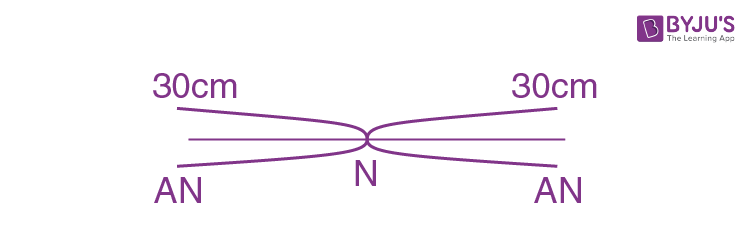$$\begin{array}{l}v = \sqrt{\frac{Y}{p}}\end{array}$$
$$\begin{array}{l}\frac{\lambda }{4} = \frac{l}{2} \Rightarrow \lambda = 2l\end{array}$$
$$\begin{array}{l}n = \frac{v}{\lambda } = \frac{l}{2l} \sqrt{\frac{Y}{p}}\end{array}$$

On substituting the value, we get the required answer.

16. Seven identical circular planar disks, each of mass M and radius R are welded symmetrically as shown. The moment of inertia of the arrangement about the axis normal to the plane and passing through the point P is: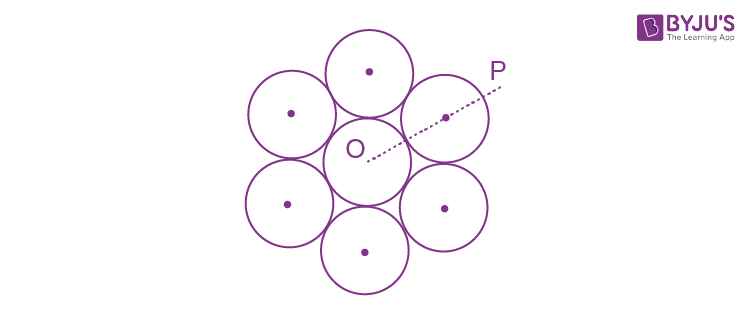a.
$$\begin{array}{l}\frac{73}{2}MR^{2}\end{array}$$

b.
$$\begin{array}{l}\frac{181}{2}MR^{2}\end{array}$$

c.
$$\begin{array}{l}\frac{19}{2}MR^{2}\end{array}$$

d.
$$\begin{array}{l}\frac{55}{2}MR^{2}\end{array}$$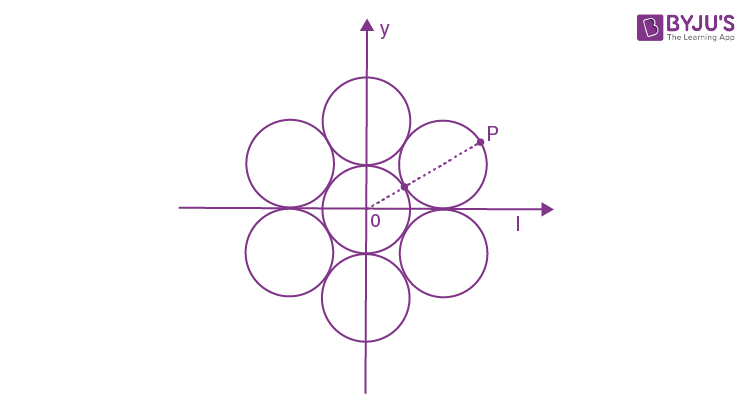$$\begin{array}{l}I_{0}=\frac{MR^{2}}{2}+6\left [ \frac{MR^{2}}{2} +M(2R)^{2}\right ]\end{array}$$
$$\begin{array}{l}I_{0}=\frac{MR^{2}}{2}+27MR^{2}\end{array}$$
$$\begin{array}{l}I_{0}=\frac{55}{2}MR^{2}\end{array}$$
$$\begin{array}{l}I_{p}=I_{0}+7M(3R)^{2}\end{array}$$
$$\begin{array}{l}I_{p}=\frac{55}{2}MR^{2}+63MR^{2}\end{array}$$
$$\begin{array}{l}I_{p}=\frac{181}{2}MR^{2}\end{array}$$

17. Three concentric metal shells A, B and C of respective radii a, b and c (a < b < c) have surface charge densities + σ, -σ and +σ respectively. The potential of shell B is:
a.
$$\begin{array}{l}\frac{\sigma }{\epsilon _{0}}\left [ \frac{b^{2}-c^{2}}{b}+a \right ]\end{array}$$

b.
$$\begin{array}{l}\frac{\sigma }{\epsilon _{0}}\left [ \frac{b^{2}-c^{2}}{c}+a \right ]\end{array}$$

c.
$$\begin{array}{l}\frac{\sigma }{\epsilon _{0}}\left [ \frac{a^{2}-b^{2}}{a}+c \right ]\end{array}$$

d.
$$\begin{array}{l}\frac{\sigma }{\epsilon _{0}}\left [ \frac{a^{2}-b^{2}}{b}+c \right ]\end{array}$$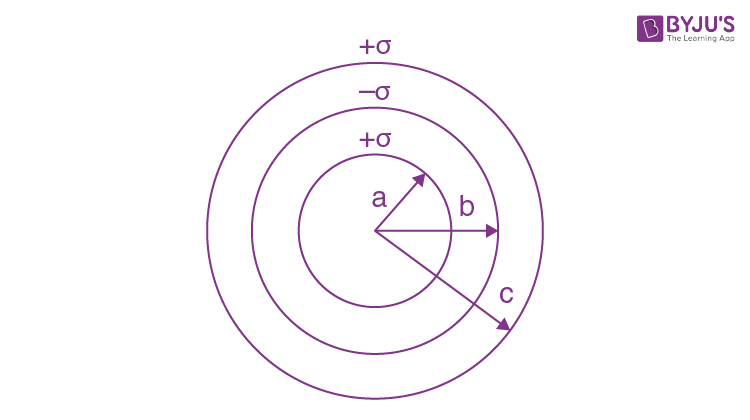$$\begin{array}{l}V_{B}=\frac{k(\sigma \times 4\pi a^{2})}{b}-\frac{k(\sigma \times 4\pi b^{2})}{b}+\frac{k(\sigma \times 4\pi c^{2})}{c}\end{array}$$
$$\begin{array}{l}\frac{\sigma }{\epsilon _{0}}\left [ \frac{a^{2}-b^{2}}{b}+c \right ]\end{array}$$

18. In a potentiometer experiment, it is found that no current passes through the galvanometer when the terminals of the galvanometer when the terminals of the cell are connected across 52 cm of the potentiometer wire. If the cell is shunted by a resistance of 5 Ω, a balance is found when the cell is connected across 40 cm of the wire. Find the internal resistance of the cell.
a. 2 Ω
b. 2.5 Ω
c. 1 Ω
d. 1.5 Ω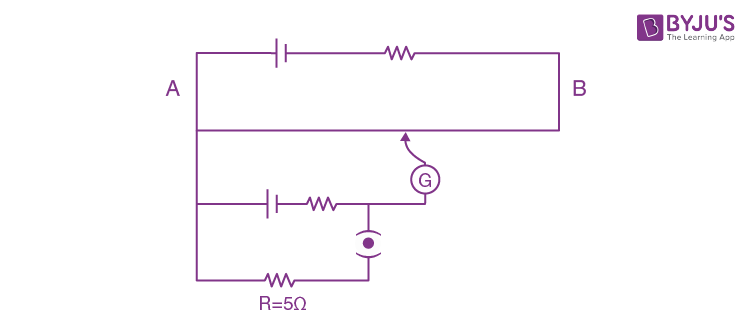When switch s is opened

E = λL1———-(I)

when switch is closed E – ir = λL2 … (II)

$$\begin{array}{l}\frac{II}{I} \frac{E-ir}{E}=\frac{L_{2}}{L_{1}}\end{array}$$

Replace i = E/(R+r)

$$\begin{array}{l}1-\frac{r}{R+r}=\frac{L_{2}}{L_{1}}\end{array}$$
$$\begin{array}{l}\frac{R}{R+r}=\frac{L_{2}}{L_{1}}\end{array}$$
$$\begin{array}{l}r=R\left ( \frac{L_{1}}{L_{2}}-1 \right )=5\left [ \frac{52}{40}-1 \right ]=5\times \frac{12}{40}=1.5\Omega\end{array}$$

19. An EM wave from air enters a medium. The electric fields are
$$\begin{array}{l}\vec{E_{1}}=E_{01}\hat{x}cos\left [ 2\pi v\left ( \frac{z}{c}-t \right ) \right ]\end{array}$$
in air and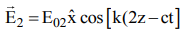in medium, where the wave number k and frequency v refer to their values in air. The medium is nonmagnetic. If εr1 refer εr2 to relative permittivities of air and medium respectively, which of the following options is correct?

a.
$$\begin{array}{l}\frac{\epsilon _{r1}}{\epsilon _{r2}}=\frac{1}{4}\end{array}$$

b.
$$\begin{array}{l}\frac{\epsilon _{r1}}{\epsilon _{r2}}=\frac{1}{2}\end{array}$$

c.
$$\begin{array}{l}\frac{\epsilon _{r1}}{\epsilon _{r2}}=4\end{array}$$

d.
$$\begin{array}{l}\frac{\epsilon _{r1}}{\epsilon _{r2}}=2\end{array}$$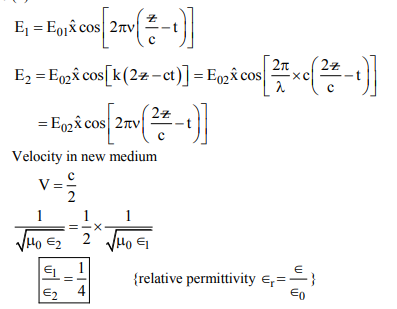20. The angular width of the central maximum in a single slit diffraction pattern is 600. The width ofthe slit is 1μm. The slit is illuminated by monochromatic plane waves. If another slit of same width is made near it, Young’s fringes can be observed on a screen placed at a distance 50 cm from the slits. If the observed fringe width is 1 cm, what is slit separation distance? (i.e., distance between the cenrtres of each slit.)
a. 75 μm
b. 100 μm
c. 25 μm
d. 50 μm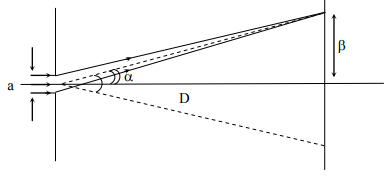2α = 600

a=1 μm

D = 50 cm

(Cond. for minima/Path diff Δx = a sin θ = n λ)

a=1 μm and θ =300 and n=1

λ = 0.5 μm

If same setup is used for YDSE

Fringe width β = λD/d = 1cm

d=0.5 x 10-6 x 0.5/0.01 = 25 μm

d = 25 μm

21. A silver atom in a solid oscillates in simple harmonic motion in some direction with a frequency of 1012/sec. What is the force constant of the bonds connecting one atom with the other? (Mole wt. of silver = 108 and Avagadro number = 6.02 x 1023 gm mole-1
a. 2.2 N/m
b. 5.5 N/m
c. 6.4 N/m
d. 7.1 N/m

Frequency

$$\begin{array}{l}f=\frac{1}{T}=\frac{1}{2\pi }\sqrt{\frac{k}{m}}\end{array}$$

K =m(2 πf))2

Mass of 1 atom

$$\begin{array}{l}m =\frac{108}{6.02\times 10^{23}}=18\times 10^{-23}gm\end{array}$$

m=18 x 10-26 Kg

k = 18 x 10-26(2π x 1012)2 = 4 π2 x 18 x 10-2

k = 7.1 N/m (π2 =10)

22. From a uniform circular disc of radius R and mass 9 M, a small disc of radius R/3 is removed as shown in the figure. The moment of inertia of the remaining disc about an axis perpendicular to the plane of the disc and passing through centre of disc is: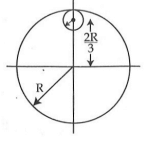a. 10 MR2
b. (37/9) MR2
c. 4 MR2
d. (40/9)MR2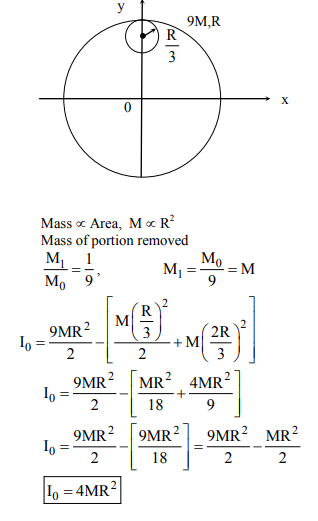23. In a collinear collision, a particle with an initial speed v0 strikes a stationary particle of the same mass. If the final total kinetic energy is 50% greater than the original kinetic energy, the magnitude of the relative velocity between the two particles, after collision, is:
a. V0/2
b. V0/√2
c. V0/4
d. √2 V0

L.M.C

mv1+mv2= mv0

V1+V2=V0———-(I)

Initial KE = (1/2)mV02

Final KE = (1/2)mV12 + (1/2)mV22

final kE = (3/2)initial kE

$$\begin{array}{l}\frac{1}{2}m\left ( V_{1}^{2}+V_{2}^{2} \right )=\frac{3}{2}\left ( \frac{1}{2}mV_{0}^{2} \right )\end{array}$$
$$\begin{array}{l}\left ( V_{1}^{2}+V_{2}^{2} \right )=\frac{3}{2}\left ( V_{0}^{2} \right )\end{array}$$
——(II)

(I)2 – (II)

2V1V2 = -(1/2) V02———-(III)

(V1-V2)2 = (V1+V2)2 – 4V1V2

(V1-V2)2 = V02+V02 =2V02

$$\begin{array}{l}V_{rel}=\left | V_{1} – V_{2}\right |=V_{0}\sqrt{2}\end{array}$$

24. The dipole moment of a circular loop carrying a current I, is m and the magnetic field at the centre of the loop is B1. When the dipole moment is doubled by keeping the current constant, the magnetic field at the centre of the loop is B2. The ratio B1/B2 is:
a. √2
b. 1/√2
c. 2
d. √3

Dipole moment μ = niA

μ = ix πR2

If dipole moment is doubled keeping current const. R2 = R1√2

Magnetic Field at center of loop

B= μ0i/2R

$$\begin{array}{l}\frac{B_{1}}{B_{2}}=\frac{R_{2}}{R_{1}}=\frac{\sqrt{2}}{1}\end{array}$$

25. The density of the material in the shape of a cube is determined by measuring three sides of the cube and its mass. If the relative errors in measuring the mass and length are respectively 1.5% and 1%, the maximum error in determining the density is:
a. 4.5 %
b. 6 %
c. 2.5 %
d. 3.5 %

$$\begin{array}{l}\rho =\frac{m}{V}=\frac{m}{l^{3}}\end{array}$$
$$\begin{array}{l}\frac{\Delta \rho}{\rho }\times 100 =\frac{\Delta m}{m}\times 100+3\frac{\Delta l}{l}\times 100\end{array}$$

= 1.5 + 3 x 1 = 4.5

26. On interchanging the resistances, the balance point of a meter bridge shifts to the left by 10 cm. The resistance of their series combination is 1 k Ω. How much was the resistance on the left slot before interchanging the resistances?
a. 550 Ω
b. 910 Ω
c. 990 Ω
d. 505 Ω

Let balancing length be L

$$\begin{array}{l}\frac{R_{1}}{R_{2}}=\frac{L}{100 -L}\end{array}$$
——–(1)

If R1 and R2 are interchanged balancing length is (L – 10)

$$\begin{array}{l}\frac{R_{2}}{R_{1}}=\frac{L-10}{110 -L}\end{array}$$
——–(2)

From equ (1) and (2)

$$\begin{array}{l}\frac{L}{100 -L}=\frac{100-L}{L-10}\end{array}$$

Hence, L2-10L = 110 x 100 +L2 – 210L

Therefore, 200L = 110 x 100

L = 55 cm

$$\begin{array}{l}\frac{R_{1}}{R_{2}}=\frac{55}{45}=\frac{11}{9}\end{array}$$

R1 + R2 = 1000 Ω

On Solving

R1 = 550 Ω

R2 = 450 Ω

27. In an a.c. circuit, the instantaneous e.m.f. and current are given by e = 100 sin 30 t and i = 20 sin(30 t – (π/4)). In one cycle of a.c., the average power consumed by the circuit and the wattles current are, respectively:
a. ((50/√2),0)
b. 50,0
c. 50,10
d. ((1000//√2),10)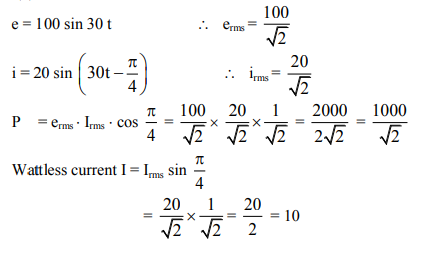28. All the graphs below are intended to represent the same motion. One of them does it incorrectly. Pick it up.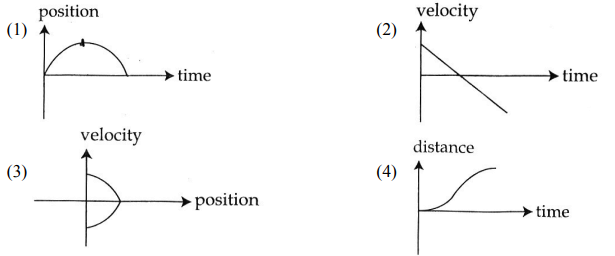If Vs t is a straight line with -ve slope acc = – ve const.

Displacement Vs time is a parabola opening downward

Only incorrect option is (4)

Correct distance Vs time graph is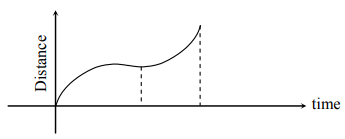29. Two moles of an ideal monoatomic gas occupies a volume V at 270C. The gas expands adiabatically to a volume 2 V. Calculate (a) the final temperature of the gas and (b) change in its internal energy.
(1) (a) 189 K (b) -2.7 kJ
(2) (a) 195 K (b) 2.7 kJ
(3) (a) 189 K (b) 2.7 kJ
(4) (a) 195 K (b) -2.7 kJ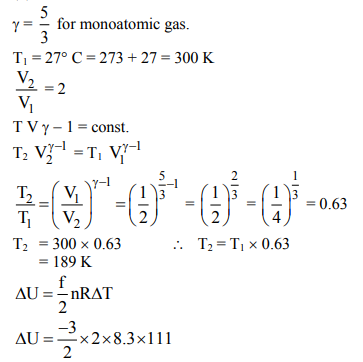ΔU = -2.76 kJ

30. A particle is moving with a uniform speed in a circular orbit of radius R in a central force inversely proportional to the nth power of R. If the period of rotation of the particle is T, then:
a. T ∝ R(n+1)/2
b. T ∝ R n/2
c. T ∝ R n/2 for any n
d. T ∝ R(n/2)+1

$$\begin{array}{l}\frac{mv^{2}}{R}=K.\frac{1}{R^{n}}\end{array}$$
$$\begin{array}{l}v^{2}=K.\frac{R}{mR^{n}}=K.\frac{1}{mR^{n-1}}\end{array}$$
$$\begin{array}{l}v=K^{‘}.\frac{1}{R\frac{(n-1)}{2}}\end{array}$$
$$\begin{array}{l}\left [ K^{‘}=\sqrt{\frac{k}{m}} \right ]\end{array}$$
$$\begin{array}{l}T=\frac{2\pi R}{v}=\frac{2\pi R\times R^{\frac{n-1}{2}}}{K^{‘}}\end{array}$$

T ∝ R(n+1)/2